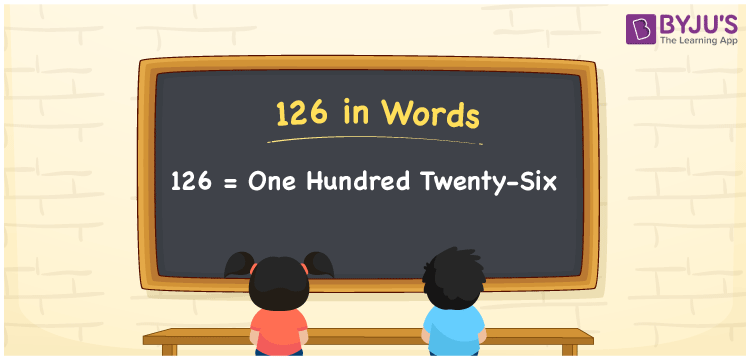# 126 in Words

We can write the number 126 in words as One hundred twenty-six. If you have written a rhyme with 126 words, you can say, “I wrote a rhyme with One hundred twenty-six words”. That means to spell the given measure or describe the numerical quantity, we will employ the word form of that particular number. In this article, you can understand how to derive the cardinal number 126 into words with the help of a place value chart effortlessly.

 126 in words One hundred twenty-six One hundred twenty-six in numerical form 126

## 126 in English Words

Generally, we use the English alphabet to write numbers in words. Thus, we express 126 in English words as “One hundred twenty-six”.## How to Write 126 in Words?

Let us derive the number 126 into words using a three-column place value chart since it contains only three digits. This can be done as given below.

 Hundreds Tens Ones 1 2 6

Here, ones = 6, tens = 2, hundreds = 1

The above digits can be expanded as:

1 × Hundred + 2 × Ten + 6 × One

= 100 + 20 + 6

= 100 + 26

= One hundred + Twenty-six

= One hundred twenty-six

Therefore, 126 in words = One hundred twenty-six

As we know, 126 is a natural number that precedes 127 and succeeds 125.

126 in words – One hundred twenty-six

Is 126 an even number? – Yes

Is 126 an odd number? – No

Is 126 a prime number? – No

Is 126 a composite number? – Yes

Is 126 a perfect square number? – No

Is 126 a perfect cube number? – No

## Frequently Asked Questions on 126 in Words

Q1

### What is the spelling of 126?

In English words, the spelling of 126 is One hundred twenty-six. Thus, we can express the number 126 as One hundred twenty-six.
Q2

### Express the value of 126 + 547 in words.

126 + 547 = 673 Thus, the value of 126 + 547, i.e., 673 in words is Six hundred seventy-three.
Q3

### How to write Rs. 126 in words on a cheque?

On a cheque, we can write Rs. 126 in words as “One hundred twenty-six rupees only”.
Test your Knowledge on 126 in Words